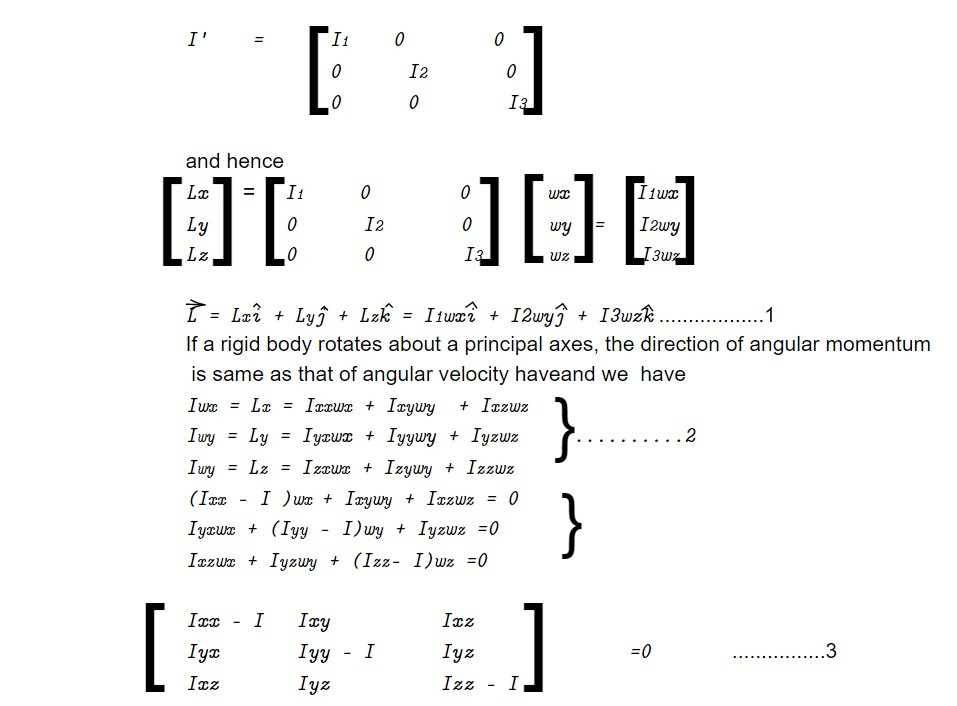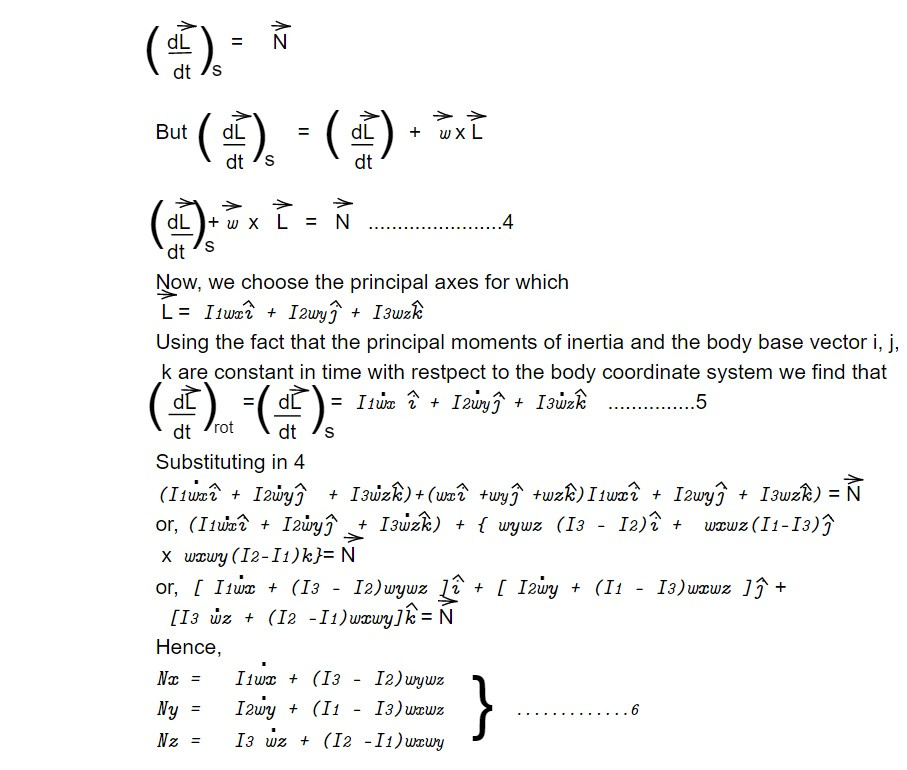# Heavy symmetrical top with one point fixed

We can select a particular set of body axes such that the off-diagonal elements vanish and the expression of inertia only contains the diagonal elements. These axes are known as the body's primary axes, and the associated moment of inertia is known as the principal moment of inertia. If we denote this form of inertia tensor by I' and I1, I2, I3 stand for the principal values.This equation gives three values of I viz, I1, I2, I3 which are desired principal moments of inertia. If a body whose I1 I2I3, it is called an asymmetrical top and if I1 = I2 = I3, it is called a spherical top.

A rigid body moving with one point stationary will do so under the influence of a torque N in a way that causes its total angular momentum to change at a rate equal to N:Equation 3 are known as Euler's equation for motion of a rigid body with one point fixed.

This note is taken from Classical Mechanics, MSC physics, Nepal.

This note is a part of the Physics Repository.

Previous topic: Angular momentum and Kinetic energy of motion about a point